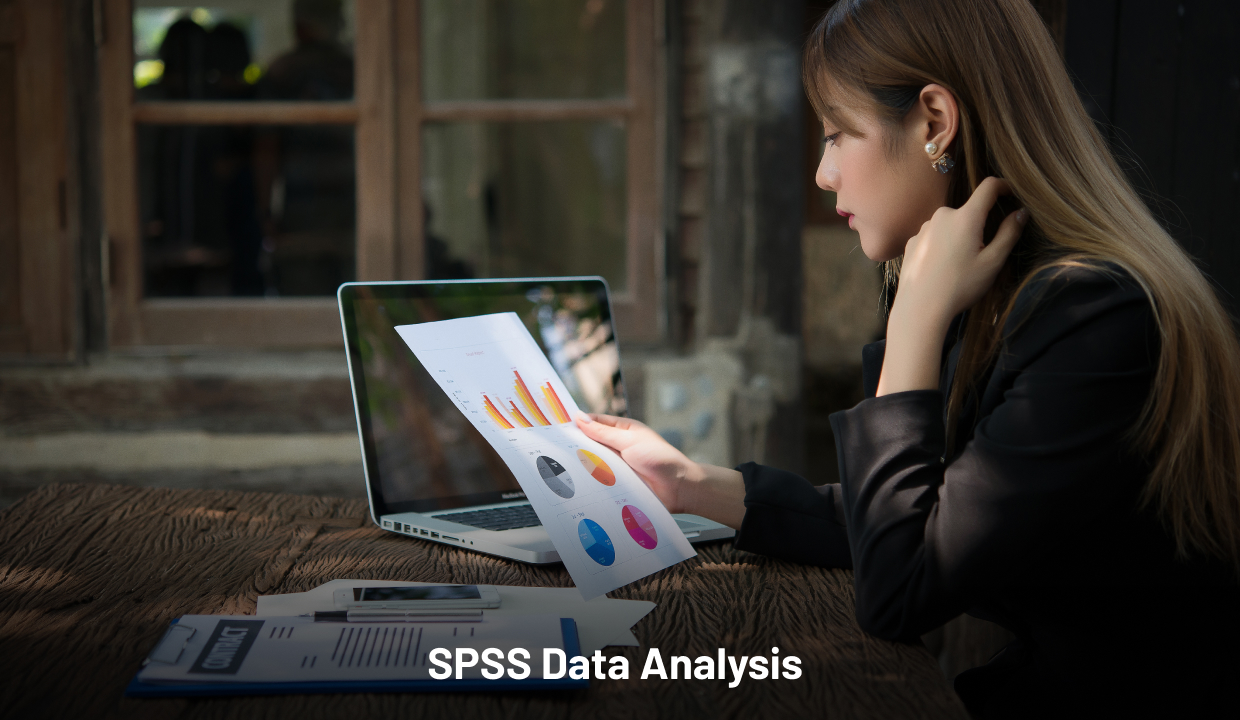## Introduction

Multivariate ANOVA (MANOVA) is the multivariate model of analysis of variance (ANOVA) that accesses multiple dependent variables simultaneously, in order to analyse the impacts of the independent variables on the dependent variables. There are multiple numbers of dependent and independent variables and the ANOVA statistical analysis is being utilised by a wide number of researchers, statisticians and students in order to conduct in depth data analysis and evaluation. The article focuses on understanding the practice of MANOVA in doing SPSS data analysis, and after understanding MANOVA in statistical tests, it would be possible to review the advantages of using MANOVA.

Through critical understanding, it is hereby possible to analyse the use of MANOVA in different statistical analytical programs in order to test the hypothesis and progress further in drawing the final conclusion of the research. The MANOVA mainly analyses the differences between three or more group means and compares it to test the hypothesis, by evaluating whether there is correlation between the dependent and independent variables or not. In ANOVA, it can be accessed with only one dependent variable and in MANOVA, it is possible to analyse the situation with multiple independent and dependent variables.

## Advantages of using MANOVA in SPSS Analysis

The statistical procedure of MANOVA provides a solution for the studies and tests the hypothesis of the research by in depth statistical analysis and critical evaluation. SPSS is being utilised to perform MANOVA by considering multiple variables, both the independent and dependent variables in the model. SPSS is the easiest statistical software, in which different statistical measures can be performed by considering the dependent and independent variables. This statistical method of MANOVA test multiple dependent variables at the same time, and by doing so, MANOVA can offer several advantages over other statistical tools.

MANOVA can detect the patterns between the multiple dependent variables, whereas ANOVA only considers only one dependent variable in the data set. Comparing the group mean values, measuring the impacts of the independent variables on the multiple dependent variables is hereby possible under MANOVA. The multivariate approach of MANOVA tests is effective for the researchers or the statisticians to represent different dependent variables efficiently through the graphs, where it is possible to analyse the changes in the dependent variables due to changes in the independent variables at a specific period of time.

The correlation coefficient can be measured with the help of MANOVA, and the researchers or the statisticians are also able to test the alternative and null hypothesis for drawing the final conclusion of the research. MANOVA is hereby beneficial for testing the statistical models with multiple dependent variables. The correlation structure between the dependent variables provides additional information to the model, which gives MANOVA the capability to test the hypothesis and analyse the impacts of the independent variables on the dependent variables. MANOVA is a one of the easy statistical model, where the researchers or the statisticians are using SPSS for in depth critical analysis and data interpretation.

## Advantages of MANOVA in Multivariate Data Analysis

MANOVA provides a scope to the researchers or the statisticians to insert multiple dependent variables, as it is a multivariate model and supports different dependent data variables for further in depth critical analysis and data interpretation. SPSS is an easy statistical software package of using different statistical measures and in this regard, most of the researchers are using MANOVA worldwide to analyse different data variables and evaluate the impacts of the independent variable on the dependent one.

It limits the joint error rate, where the researchers can avoid errors in the data set and progress further for better data management and analysis. Data handling becomes easier with the
SPSS help, where it is possible for the researchers or the data analysts to insert the data and handle the large data set by avoiding error value and mission variables in the data set. This further improves the quality of the data variables for performing MANOVA. As MANOVA considers multiple dependent variables, the joint probability of rejecting a true null hypothesis increases with the additional test. The use of MANOVA is helpful for the researchers where the error rate equals the significance level. The access of patterns between the multiple dependent variables is also different from other statistical tests in SPSS, where the factors in the model can affect the relationship between the dependent variables present in the data set, rather than considering only one dependent variable.

MANOVA can also detect the pattern of changing the dependent variables due to the significant changes in the independent variables in the data set. Greater statistical power is another advantage of MANOVA, where it is possible for the researchers or the data analysis to perform the statistical analysis accurately and identify the value of correlation coefficient for evaluating the positive or negative changes in dependent variables due to the changes in the independent variables in the data set. it can also shows the differences in
ANOVA tests between the variables as well as the researchers can improve their chances of finding what changes as a result of the experimental treatment in the data set. The statistical analysis is also possible to detect whether the groups differ along a combination of the variables.

##Conclusion

It can be concluded that MANOVA is useful for the data analysis or the researchers to test the research hypothesis, in case of multiple dependent variables. Through this reliability analysis, the data analysts can analyse the impacts of the multiple independent variables on the multiple dependent variables in the data set.